# Porous Media Simulation Guide For FSAE Radiator

#81

After focusing more time on getting the porous media results to match the simulated radiator results, I have found some areas that are unclear. The first calculations of the Darcy Forchheimer coefficients have been recorded using alpha and beta values from the curve fit polynomial. The problem lies in the fact that removing the Y-Intercept changes the alpha and beta values in the equation. Doing so results in the following equation:

## y = 4,39x2 + 22,922x

Where:

Alpha = 22.922

Beta = 4.39

This results in the following coefficients for the porous media

Darcy Coefficient without Y-intercept 72895531.88
Forchheimer Coefficient without Y-intercept 403.59

I then ran two straight on porous media tests with the velocity and pressure drop data to relate to. This test was done with the following settings

• 35 deg c
• 10.121 m/s

Average Pressure drop assumed

Pressure before = 869 Pa

Pressure after = -2.5 Pa

Pressure drop = 871.5 Pa

Without y- intercept value - Pressure

Without Y- intercept value - Velocity

Velocity at face = 11 m/s

Results of Straight on Porous media without Y- Intercept VS actual data

Straight on Porous media without Y- Intercept Actual Data
Velocity m/s Pressure Drop Pa Velocity m/s Pressure Drop Pa
11 m/s 871.5 10.121 679
Difference 0.879 192.5

With y- intercept value

The second radiator run was done using the same velocity settings as the first run (10.121 m/s). However this time the curve fit equation was slightly changed by adding the Y-Intercept selection. This gives the full equation, and with the Y- intercept ignored a new alpha and beta result. Which results in the equation.

## y = 4,1342x2 + 26,185x - 8,7

This gives the following coefficients

Darcy Coefficient 83272380.35
Forchheimer Coefficient 380.07

Pressure before = 879 Pa

Pressure after = -2.5 Pa

Pressure drop = 883.4 Pa

With Y- intercept value - Pressure

With Y- intercept value - Velocity

Velocity at face = 11m/s

Results of Straight on Porous media WITH Y- Intercept VS actual data

Straight on Porous media WITH Y- Intercept Actual Data
Velocity m/s Pressure Drop Pa Velocity m/s Pressure Drop Pa
11 m/s 883.4 10.121 679
Difference 0.879 204.4

Next I decided to simulate the radiator at the 50 degree angle it will be positioned at on the car. The results from these simulations provided better coherence to the actual data, which makes me think this was taken into account with the real radiator simulations. The first sideways radiator run was done using the same alpha and beta results WITH the Y-Intercept values kept and again using the same coefficients.

Darcy Coefficient 83272380.35
Forchheimer Coefficient 380.07

Pressure before = 625 Pa

Pressure after = -12 Pa

Pressure drop = 637 Pa

50 deg angle - With Y- intercept value - Pressure

50 deg angle - With Y- intercept value - Velocity

Velocity at face ~ 10.8 m/s

Results of 50 deg angle Porous media WITH Y- Intercept VS actual data

50 deg angle Porous media WITH Y- Intercept Actual Data
Velocity m/s Pressure Drop Pa Velocity m/s Pressure Drop Pa
10.8 m/s 637 10.121 679
Difference 0.679 42

Now there are some questions along with my setup of these tests.

1. In my straight on simulations, the velocity cutting plane shows a large increase along the edges of the radiator. Is this normal or did i set something up wrong in my meshing or simulation?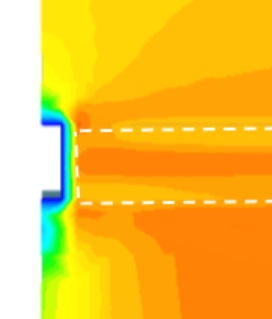1. In the 50 deg angle porous media i setup a housing so that the square porous media would not get cut off by the bounding box. The pressure and velocity cutting planes show disturbance in these areas and i’m not sure how it will affect the results

2. The data to be selected can vary a lot. The tilted radiator is, in my opinion, very close to my actual data, and if I simply collect my simulated data from different points i can make it match almost exactly. Sampling from this location gives me -35Pa after the radiator wich makes my new pressure drop 660 Pa. How accurate must this actually be? there are so many variables. I also have 10.121 m/s as inlet velocity not face velocity. This will further change the measurements.

#82

This velocity profile is interesting. I’d say it’s likely related to these big surface cells that you have. y+ should be quite high here and the BL profile is not being captured appropriately. Remember that wall function bridges (models) the cell center to the surface.
The rest of the boundaries look normal because they’re set to slip walls.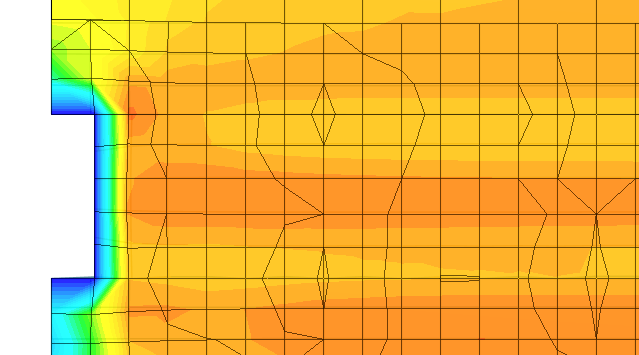#83

Oh, i thought i didnt need a BL at all because its a porous media? I had everything set to slip walls (even the radiator housing) because i want the data from just the porous media. I wanted to do a BMB with only the media but that didnt mesh well. Also do you use the free standing zone faces?

With this off the radiator looks like this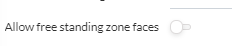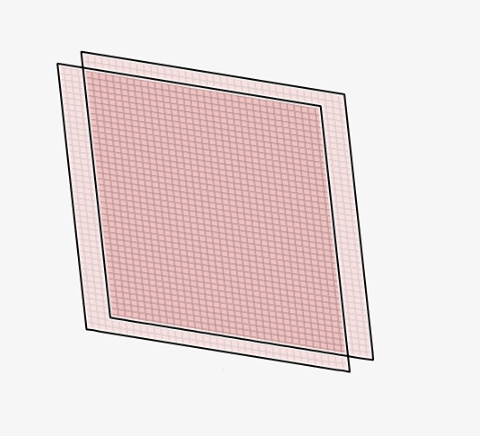With this option ON it looks like a normal volume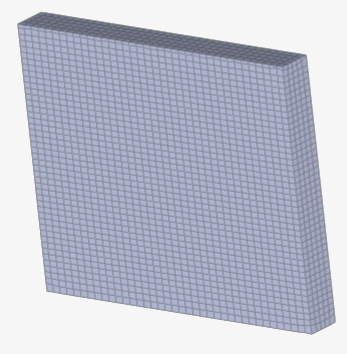also speaking of velocity Profiles looking funny, on the 50 deg media test, i added a housing that tries to influence the flow as llittle as possible. The left arrow is an area of concern for me because this is an anisotropic media and the flow seems to be moving not so parallel with the medium. Is this my error or normal?

#84

I have also tried a run with the whole setup, porous media, radiator exhaust into fans with MRF zones. The fans appear to be working but my particle traces dont seem to be going through the porous media. The settings are the exact same as the closed wind tunnel shown above. Any idea on whats going wrong?